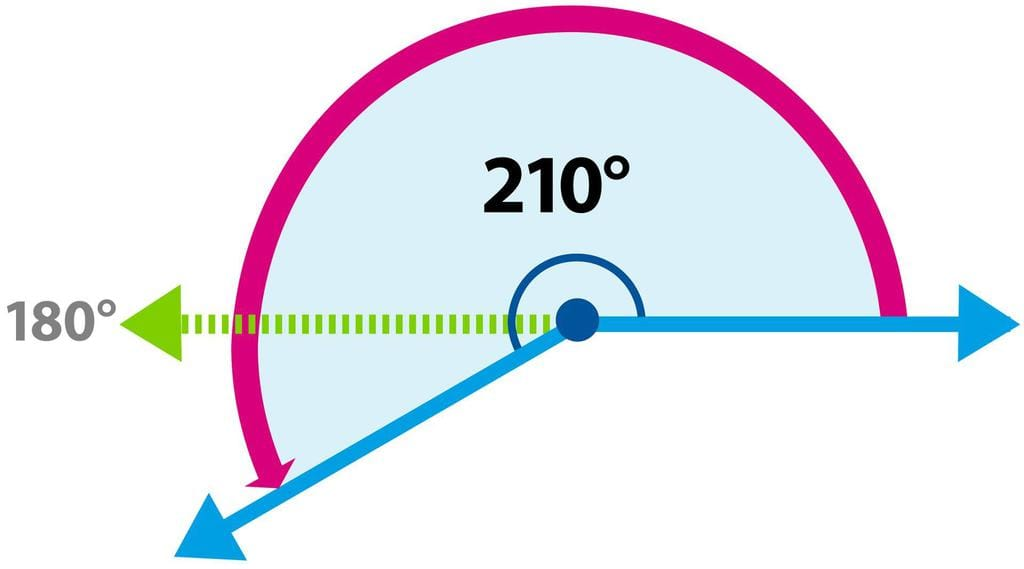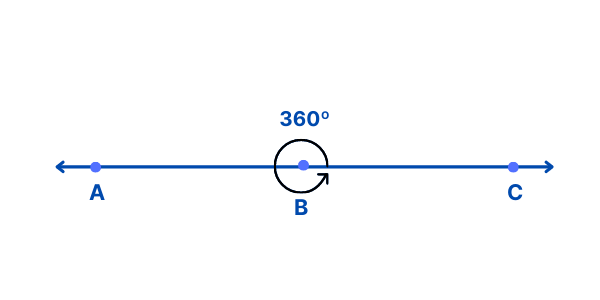Test: Geometry- 1

# Test: Geometry- 1

Test Description

## 10 Questions MCQ Test Mathematics for Class 4 | Test: Geometry- 1

Test: Geometry- 1 for Class 4 2023 is part of Mathematics for Class 4 preparation. The Test: Geometry- 1 questions and answers have been prepared according to the Class 4 exam syllabus.The Test: Geometry- 1 MCQs are made for Class 4 2023 Exam. Find important definitions, questions, notes, meanings, examples, exercises, MCQs and online tests for Test: Geometry- 1 below.
Solutions of Test: Geometry- 1 questions in English are available as part of our Mathematics for Class 4 for Class 4 & Test: Geometry- 1 solutions in Hindi for Mathematics for Class 4 course. Download more important topics, notes, lectures and mock test series for Class 4 Exam by signing up for free. Attempt Test: Geometry- 1 | 10 questions in 15 minutes | Mock test for Class 4 preparation | Free important questions MCQ to study Mathematics for Class 4 for Class 4 Exam | Download free PDF with solutions
 1 Crore+ students have signed up on EduRev. Have you?
Test: Geometry- 1 - Question 1

### A flag is always parallel to the ground

Detailed Solution for Test: Geometry- 1 - Question 1

A flag is always perpendicular to the ground, not parallel.

Hence, False.

Test: Geometry- 1 - Question 2

### Find the angle of acute angle.

Detailed Solution for Test: Geometry- 1 - Question 2

An acute angle is an angle that measures less than 90°. It can be measured using a protractor. For example, 35°, 60°, 72°, and so on are all acute angles.

Test: Geometry- 1 - Question 3

### Stumps of a wicket is example of

Detailed Solution for Test: Geometry- 1 - Question 3

Stumps of a wicket is an example of perpendicular.

Test: Geometry- 1 - Question 4

A line cannot be drawn fully on a

Detailed Solution for Test: Geometry- 1 - Question 4

A line cannot be drawn on a paper. We can only draw a line segment on a paper. A line extends indefinitely in both directions and therefore we cannot draw a line on a paper. Whereas a line segment is of finite length.

Test: Geometry- 1 - Question 5

What the angle of reflex angle?

Detailed Solution for Test: Geometry- 1 - Question 5

A reflex angle is any angle that is more than 180 degrees (half circle) and less than 360 degrees (full circle). A reflex angle will always have either an obtuse or an acute angle on the other side of it.Test: Geometry- 1 - Question 6

Ruler is a example of _________ angle.

Detailed Solution for Test: Geometry- 1 - Question 6

Ruler is an example of straight  angle.

In Maths, a straight angle is an angle equal to 180 degrees. It is called straight because it appears as a straight line.Test: Geometry- 1 - Question 7

180 is a _________ angle.

Detailed Solution for Test: Geometry- 1 - Question 7

180 is a straight angle.Test: Geometry- 1 - Question 8

Find the complete angle

Detailed Solution for Test: Geometry- 1 - Question 8

A full angle, also called a complete angle, and 360 is a complete angle.Test: Geometry- 1 - Question 9

Corner of room is _________ angle

Detailed Solution for Test: Geometry- 1 - Question 9

Corner of room is right angle

Test: Geometry- 1 - Question 10

All perpendicular lines are _________ lines

Detailed Solution for Test: Geometry- 1 - Question 10

All perpendicular lines are intersecting lines

## Mathematics for Class 4

17 videos|68 docs|32 tests
 Use Code STAYHOME200 and get INR 200 additional OFF Use Coupon Code
Information about Test: Geometry- 1 Page
In this test you can find the Exam questions for Test: Geometry- 1 solved & explained in the simplest way possible. Besides giving Questions and answers for Test: Geometry- 1, EduRev gives you an ample number of Online tests for practice

## Mathematics for Class 4

17 videos|68 docs|32 tests